Next: 3 Data Quality Flag Up: 2.3.2 High-Dispersion Mode Previous: 2.3.2.1 Resolution Along the

2.3.2.2 Resolution Perpendicular to the Dispersion

The spatial resolution has been determined by analyzing the spectra of high-dispersion standard stars. The FWHM of several pairs of large and small-aperture line-by-line images were measured at five sample positions (viz., 134, 258, 384, 507, and 615). For each sample position, a three pixel wide average cross-cut perpendicular to the dispersion was taken and the widths of the orders measured using the gaussian fitting routine. The results for each image were in good agreement, so we averaged the results to yield a set of spectral widths for each aperture as a function of order number and sample position. The differences in telescope focus between the images were kept small so as to minimize the effect of focus on the resolution measurements (Pérez et al. 1990). The database of spectra used for each camera contains a combination of optimally exposed images for the central orders and overexposed (in the central orders only) images for the extreme orders. The spatial resolution data and the one-sigma error bars for each sample position are plotted as a function of order number. The small-aperture data are horizontally offset to the left of the large-aperture data by half an order for clarity. A seventh-order polynomial fit to the data is also provided.

LWP - Spatial resolution measurements of the FWHM are plotted in Figures 2.28-2.32. The spatial resolution for sample position 384 is approximately 3.5 pixels FWHM at order 69 and decreases to 2.3 pixels at order 80 where it is roughly constant for the remaining orders. The spatial resolution degrades as one moves towards smaller sample positions and improves slightly (as compared with sample position 384) above order 90 for sample position 507. Small-aperture resolution shows an average improvement (over all orders and sample positions) of 4.6% over the large aperture. This difference is most apparent between orders 80 through 100 and at the smaller sample positions where it is as much as 8% for sample position 134. Unfortunately, no LWP high-dispersion spatial resolution studies could be found for IUESIPS data to compare against the NEWSIPS results.

LWR - Figures 2.33-2.37 show spatial resolution measurements of the FWHM plotted as a function of order number. The resolution trends for sample positions 134 through 384 are quite similar. The FWHM is approximately 3.0 pixels for order 69 and linearly decreases to 2.4 pixels at order 80 where it remains fairly constant for the remaining orders. For sample position 507, the FWHM is around 3.2 pixels for order 69 and linearly decreases to 2.6 pixels at order 80 where it then gradually decreases to 2.3 pixels at order 123. The behavior for sample position 615 demonstrates a rapid decrease in FWHM from 3.8 pixels at order 69 to 2.7 pixels at order 95 where it then gradually decreases to 2.3 pixels at order 120. The small-aperture resolution shows an improvement of approximately 4.7% over the large aperture.

The IUESIPS FWHM measurements obtained by Cassatella et al. (1981) using WAVECAL images are somewhat inconclusive. Their data only includes 5 orders (71, 73, 77, 81, and 90) and no mention was made of the sample positions at which these measurements were taken. Their numbers range from 3.5 pixels at order 71 to 2.7 pixels at order 90; values which are around 10% higher than the corresponding NEWSIPS FWHM measurements. The trends seen in the 2-D contour plots made by de Boer et al. (1983) are in good agreement with the NEWSIPS results. They show that for the central sample positions (i.e., 384) the FWHM starts out at 3.1 pixels at low order numbers and decreases to 2.8 pixels towards the center of the camera (e.g., order 90). The slight degradation in resolution seen in the central orders of Figure 2.35 is also apparent in the de Boer plots.

SWP - Spatial resolution measurements of the FWHM are plotted in Figures 2.38-2.42. The resolution trends as a function of order number are, in general, the same for every sample position. The FWHM is around 4 pixels at order 66 (long wavelengths) and decreases to approximately 2 pixels near order 100 (short wavelengths). Unlike the indications from previous IUESIPS studies (e.g., Bianchi (1980), Schiffer (1980), and Cassatella et al. (1981)), this decrease is not linear with order number. A plateau of around 3.0 pixels FWHM occurs between orders 75 and 85. This trend is confirmed by the analysis of de Boer et al. (1983), which displayed the order widths using 2-D contour plots. The FWHM remains fairly constant above order 100 for sample positions 258 and 384. At these sample positions, the higher orders (100 and above) are well away from the edge of the camera. The more extreme sample positions (i.e., 134 and 507) show an edge effect as the resolution dramatically worsens above order 100. The best spatial resolution occurs near sample position 384 and worsens slightly as one moves towards smaller sample positions (i.e., shorter wavelengths within an order). Differences in resolution between the large and small apertures are small. The small aperture shows an average improvement (over all orders) of 2.4% in resolution over the large aperture.

As is the case with the low-dispersion resolution studies, the NEWSIPS values show an improvement over IUESIPS measurements. Schiffer (1980) quoted FWHM values of 3.5 pixels for order 75 and 2.4 pixels for order 105. The NEWSIPS results for those orders are 3.3 pixels and 2.1 pixels, respectively. Analysis by de Boer et al. (1983) showed the best resolution of 2.4 pixels FWHM occurring near the center of the camera. The NEWSIPS results indicate a FWHM of 2.0 pixels in this same area (sample position 384). Also, Bianchi (1980) expressed FWHM as a function of order number, regardless of camera, according to the following formula: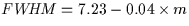where m is order number and the FWHM is in pixels. Thus, for order 71, this indicates a FWHM of 4.4 pixels, a figure that is almost 20% higher than the NEWSIPS average measurement for that order.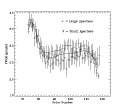Figure 2.28: LWP high-dispersion spatial resolution for sample position 134. Small-aperture data is horizontally offset to the left of the large-aperture data by half an order.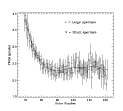Figure 2.29: LWP high-dispersion spatial resolution for sample position 258. Small-aperture data is horizontally offset to the left of the large-aperture data by half an order.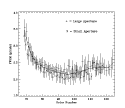Figure 2.30: LWP high-dispersion spatial resolution for sample position 384. Small-aperture data is horizontally offset to the left of the large-aperture data by half an order.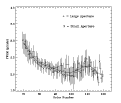Figure 2.31: LWP high-dispersion spatial resolution for sample position 507. Small-aperture data is horizontally offset to the left of the large-aperture data by half an order.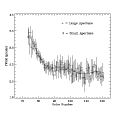Figure 2.32: LWP high-dispersion spatial resolution for sample position 615. Small-aperture data is horizontally offset to the left of the large-aperture data by half an order.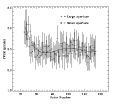Figure 2.33: LWR high-dispersion spatial resolution for sample position 134. Small-aperture data is horizontally offset to the left of the large-aperture data by half an order.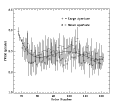Figure 2.34: LWR high-dispersion spatial resolution for sample position 258. Small-aperture data is horizontally offset to the left of the large-aperture data by half an order.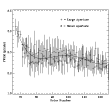Figure 2.35: LWR high-dispersion spatial resolution for sample position 384. Small-aperture data is horizontally offset to the left of the large-aperture data by half an order.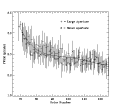Figure 2.36: LWR high-dispersion spatial resolution for sample position 507. Small-aperture data is horizontally offset to the left of the large-aperture data by half an order.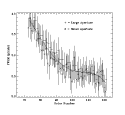Figure 2.37: LWR high-dispersion spatial resolution for sample position 615. Small-aperture data is horizontally offset to the left of the large-aperture data by half an order.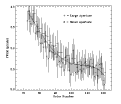Figure 2.38: SWP high-dispersion spatial resolution for sample position 134. Small-aperture data is horizontally offset to the left of the large-aperture data by half an order.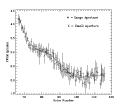Figure 2.39: SWP high-dispersion spatial resolution for sample position 258. Small-aperture data is horizontally offset to the left of the large-aperture data by half an order.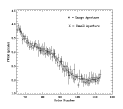Figure 2.40: SWP high-dispersion spatial resolution for sample position 384. Small-aperture data is horizontally offset to the left of the large-aperture data by half an order.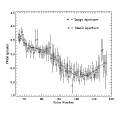Figure 2.41: SWP high-dispersion spatial resolution for sample position 507. Small-aperture data is horizontally offset to the left of the large-aperture data by half an order.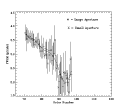Figure 2.42: SWP high-dispersion spatial resolution for sample position 615. Small-aperture data is horizontally offset to the left of the large-aperture data by half an order.Next: 3 Data Quality Flag Up: 2.3.2 High-Dispersion Mode Previous: 2.3.2.1 Resolution Along the
Karen Levay
12/4/1997